# Deep Learning Papers review — Universal Adversarial Patch

In this article, I’ll initially be discussing about generating adversarial images and then I’ll slowly steer the discussion towards an interesting paper published by researchers at Google Brain about an Adversarial Image Patch (https://arxiv.org/pdf/1712.09665.pdf). This paper presents a generic image patch, which when added to images would cause any Neural Network to misclassify them. The authors of the paper themselves have demonstrated this through a youtube video :

Let’s first find out why such adversaries can be formed in the first place.

#### Weaknesses of Neural Networks

Deep Neural Networks have certainly been producing “high accuracy” results for object recognition lately. Yet, one can make a Neural Net misclassify an image with minimal perturbations. Let’s take a look at the possible reasons :

• Deep Neural Nets are trained on a fixed set of data and hence, transformations to the input signals like translation or rotation could make it misclassify. This also means that, a small amount of noise added to the input signal could cause it to misclassify. For eg., adding a small amount of noise to an input image could cause a Neural Network to misclassify the image even though a human eye wouldn’t perceive any change in the image. This image would give you an idea :

[There was recently some work on Capsule Networks by Geoff Hinton, which are invariant to image transformations. Yet, capsules are vulnerable to other type of adversarial attacks. And even Convnets are more or less scale and transformation invariant ]

• Also, today’s Deep Learning based classifiers are also mostly piecewise linear. Even the most popular activation functions like ReLu (and it’s variants) are part by part linear. Other activation functions like Sigmoid and Tanh are ruled out here as they cause issues such as the “Vanishing Gradient Problem”. Although Neural Networks are “non-linear classifiers”, they attain this so called nonlinearity through multiple “linear” regions

These weaknesses of Neural Nets gave rise to an entire field called “Adversarial Deep Learning” (in general “Adversarial Machine Learning” for any type of input signal)

#### Generating Adversarial Images

Generating adversarial images to fool a Neural Network classifier isn’t a new problem. There have been a lot of proposed methods in the past to generate adversarial examples. The simplest way to do this would be to change the value of individual pixels of the image until the probability of a new class is maximized. Mathematically,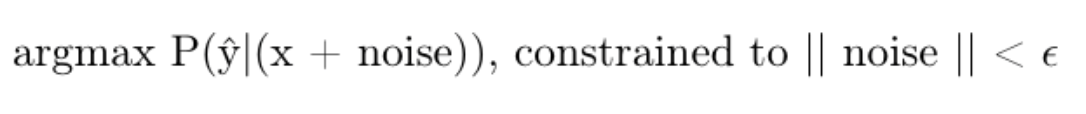Simple mathematical equation to build adversarial images

(Most researchers usually replace the above probability term with the log probability)

There are also gradient based iterative methods like, Fast gradient sign method (FGSM), Iterative gradient sign method and Iterative Least-likely Class Method to produce adversarial examples. These methods primarily use the gradient of the cost (J) of the output class with respect to the input image, to iteratively change the input image based on the gradient. Let’s take a look at the mathematical equation of FGSM :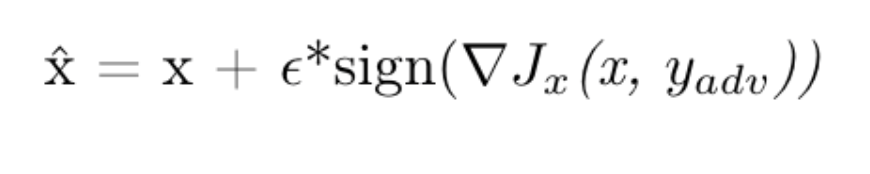FGSM

In a nutshell, FGSM iteratively increases the input signal by a small amount in the direction of the gradient of the cost with respect to the input.

Apart from the above techniques, of course there are the popular GANs (Generative adversarial networks) to generate adversarial images.

While the above methods generate satisfactory adversarial examples, they aren’t robust enough to work on equivalently transformed images. This paper titled “Foveation-based Mechanisms Alleviate Adversarial Examples” by Luo et. al, shows that the above adversarial examples fail when they are cropped along the object of interest (Foveated). This is because, Convnets are robust towards scaling and translation. But, such a transformation rule doesn’t apply to the noise or perturbation added to the image, i.e., the perturbations aren’t robust enough to fool the Convnet even after the image getting transformed. Another paper titled “NO Need to Worry about Adversarial Examples in Object Detection in Autonomous Vehicles”, has almost the same intent.

So, is it even possible to produce a robust set of adversarial images ? Well, there have been some interesting papers lately that discuss about producing robust adversarial examples. We’ll take a look at some of them :

We’ll mainly be looking into the first 2 papers.

#### Expectation Over Transformation (EOT)

The work from the first paper (i.e., Synthesizing robust adversarial examples), produces adversarial examples that are robust enough to “fool” a Neural Network classifier under most image transformations. Essentially, what happens here is that, the Expected probability of a class is maximized, over all possible transformation functions (t ~ T), with a constraint on the Expected effective distance between the transformed original and transformed perturbed image. Let’s try to understand what that means.

In EOT, the given image is first made adversarial using one of the above mentioned methods. Now, we define a transformation space ‘T’, which houses transformations like rotation, scaling, translation and so on. Then, we calculate the expectation of the log probability of our desired class label. This is what it looks like mathematically :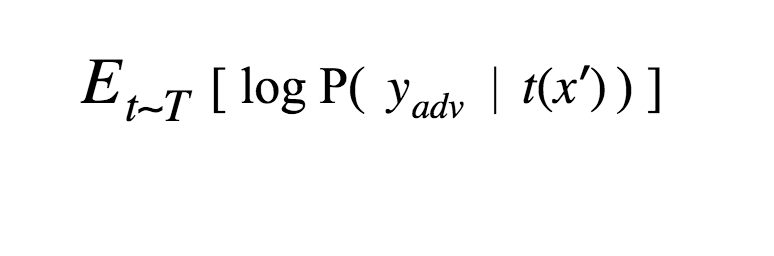Expected log probability of the desired class given the transformations

We then try to maximize this expected probability under the constraint that the Expected effective distance between the transformed original and the transformed perturbed image is less than some value ‘ε’. So, by considering the expected probability (or log probability), we’re accounting for all the transformations present in the Transformation space. And the constraint is to ensure that the generated images are as close as possible to the original transformation. This is what the final equation looks like :

#### Adversarial Patch

From the video above, it’s clear that we are hunting for a “Universal” image patch, which when added to any image will make a Neural Network misclassify the image. For this, an operator`A()` is first defined. The operator `A` takes in a patch, an image, co-ordinates in the image (to place the patch) and transformations like translation, rotation and scaling to be applied on the patch.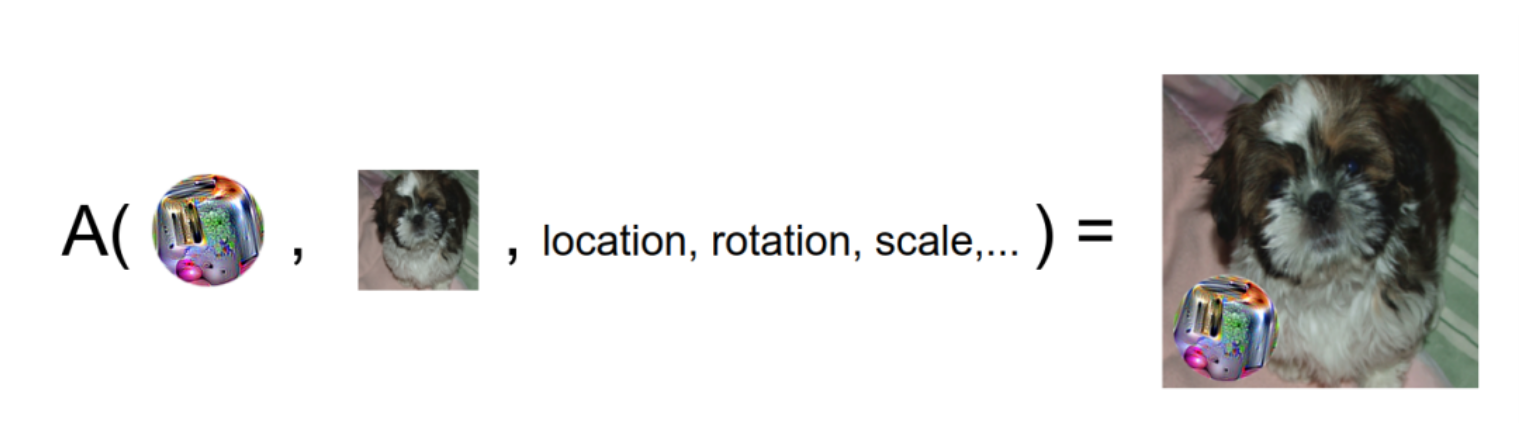An intuition behind the operator ‘A’

To find the optimal patch, Expectation over Transformation is used for a given label to maximize the probability of misclassification. Mathematically, it looks like this :

The original paper used “Toaster” as the adversarial class and the final patch looked like this :

One limitation about this adversarial patch is that, you can’t fool object detection models (models that recognize various object in an image). For eg., I recently tried to upload an image with this patch onto Facebook (:P). Since Facebook lists all the predictions about the image in the `alt` attribute of the `img` tag that houses it, you can check its predictions as soon as you upload the image. Here’s what I tried :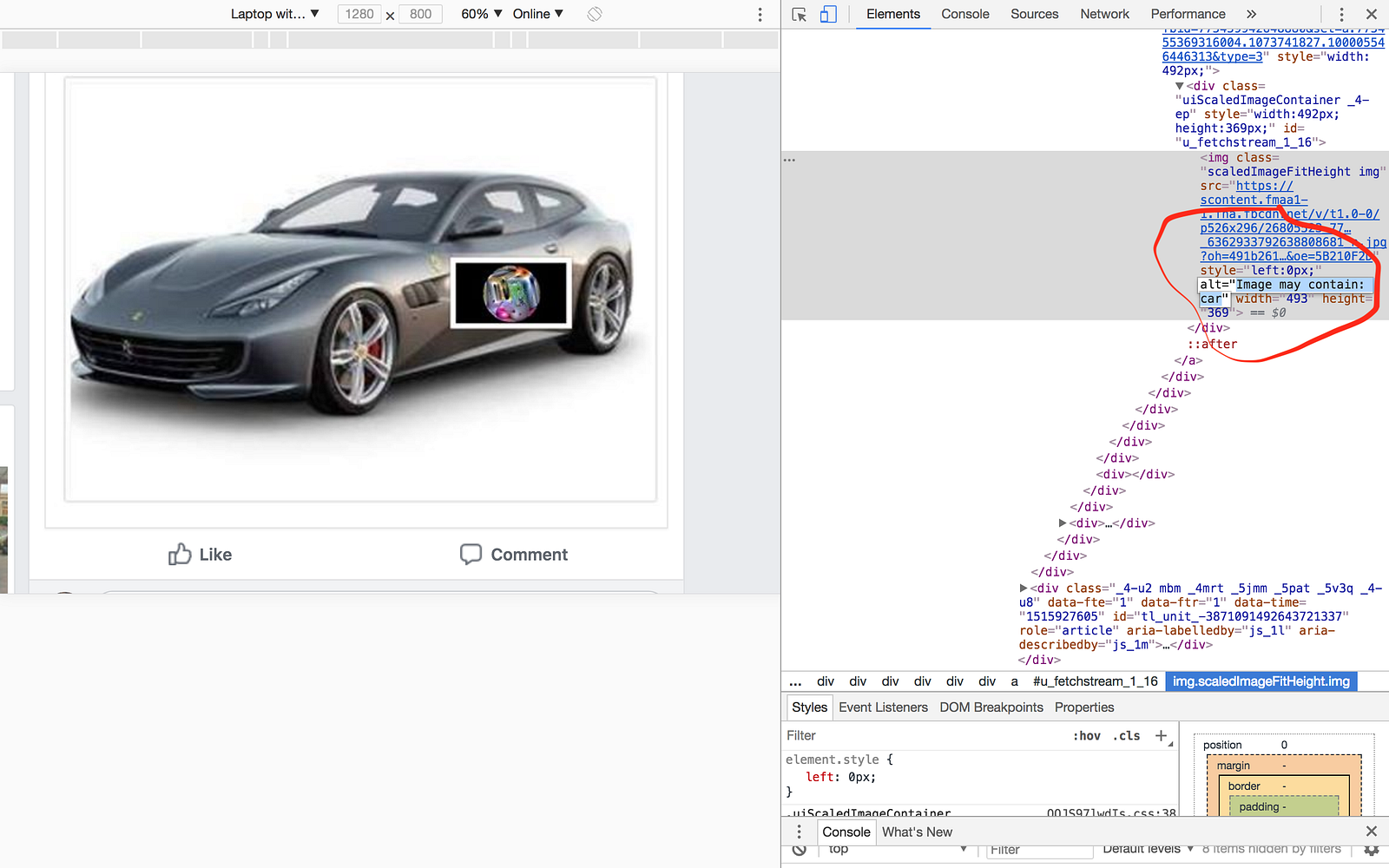Left : My facebook post, Right : Chrome Dev tools

(:P)

[The 3rd paper in the list above, i.e., “Towards Imperceptible and Robust Adversarial Example Attacks against Neural Networks came out just about a week back. In that paper, they’ve taken into account the human perceptual system while generating adversarial examples]

#### Summary

• Generating adversarial content : We essentially increase the probability of misclassification by repeated addition of noise. Some popular techniques like FGSM use the sign of the gradient of the cost to add noise
• Weakness : Those methods aren’t robust enough to “fool” a Neural Network when the input perturbed image gets transformed (arxiv:1511.06292 and arxiv:1707.03501)
• Expectation over transformation : We first generate an adversarial image using one of the above methods. Then, we maximize the expected log probability of a class, for a given transformation of the perturbed image. This expectation is over all the transformations in the transformation space ‘T’
• Adversarial patch : Defines an operator ‘A’ that applies a patch onto the given image. Then, Expectation over transformation is used to maximize the log probability of a new class, under the constraint that it doesn’t deviate too much from the starting patch